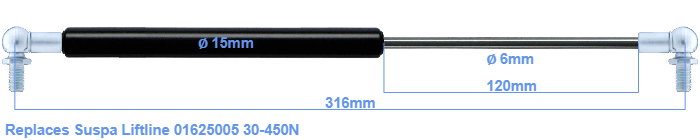# Replacement for Suspa Liftline 01625005 30-450N

\$31.74

Replacement gas spring for the Suspa Liftline 01625005 30-450 Newton. This fits a (possibly already present) ball with a diameter of 10mm. A corresponding ball is included. Brand: Stabilus Industry Line.
 Force Choose an option30 Newton40 Newton50 Newton60 Newton80 Newton100 Newton120 Newton140 Newton150 Newton160 Newton180 Newton200 Newton220 Newton240 Newton250 Newton260 Newton280 Newton300 Newton320 Newton340 Newton350 Newton360 Newton380 Newton400 Newton420 Newton440 Newton450 NewtonClear
This gas spring is also known as 16-1 016 25005, 16-1-154-124-A246-B246.The bore of the house of this replacement gas spring is 15 mm. The rod has a diameter of 6 mm. The stroke of the gas spring is 120 millimeter. The total length of this replacement gas spring is 316 mm. This is the total distance between the centers of the two mounting parts. Without the mounting parts this gas spring is 272 mm long (thread to thread). This gas spring (01625005) has a force of 30-450 Newton. This is not an original gas spring produced by Suspa Liftline, but it can replace a Suspa Liftline gas spring. This gas spring was produced by Stabilus subsidiary Hahn with the brand Stabilus Industry Line. Nevertheless, the dimensions and force are equal.
Category: